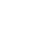# How To Multiply In Excel

H

How to multiply in Excel is an easy thing to do. There are many ways to do so, so let us learn about them.

Microsoft Excel is widely popular and is used by people from all backgrounds. Whether you are a student or a working professional, Excel helps you in several things and you use it for small and significant calculations.

Some calculations are easy to do and some are not so and I am sure multiplication is something that most people get stuck at. In comparison to other computations, such as finding the average, calculating age, multiplication is probably the one that most people struggle with. If you want to learn the technique of multiplying in Excel then this article is just for you. This skill would help you throughout your life.

## How To Multiply In Excel

In Excel, you can actually multiply in three different ways and that too in just a few seconds. Let us take a look at each one of them.

### 1. Multiply numbers in Excel using a basic formula

With these two methods, you can quickly multiply numbers in a single cell as well as in multiple cells using a basic formula.

#### Multiply numbers in a single cell

1. Open Microsoft Excel, and select any empty cell.

2. In the selected cell, type an equal sign (=) followed by the numbers you want to multiply with an asterisk between them.

3. Press “Enter”. Your equation will disappear, and the answer will be displayed.

#### Multiply the numerical content of different cells

When you want to multiply two or more cells in Excel, follow these steps:

1. Open the Microsoft Excel spreadsheet containing the numbers you want to multiply and type an equal sign (=) in an empty cell.

2. Click on the first cell you want to multiply, and the cell name will appear next to the equal sign. Type an asterisk next to the cell name that appears.

3. Click on the second cell you want to multiply to add it to the formula. Repeat the asterisk and click on additional cells to add more factors to your equation.

4. Press the “Enter” key, and the answer will appear.

### 2.Multiply numbers in Excel using the PRODUCT formula

You can also use the PRODUCT formula to multiply up to 255 values at once.

1. Open Microsoft Excel and select any empty cell. Type an equal sign, followed by the word “PRODUCT” in all capital. Add an opening parenthesis.

2. To multiply individual cells, type the names of the cells and separate them using commas with no spaces.

3. To multiply a series of cells, enter a colon between two cell names to indicate that all cells within that range should be multiplied. For example, “=PRODUCT(A2:A5)” indicates that cells A2, A3, A4, and A5 should be multiplied.

4. In case you want to add a number to the equation, type a comma and then that number.

5. Once you finish entering the contents of your formula, place a closing parenthesis at the end and press the “Enter”. The equation answer will appear in the cell.

### 3. Multiply numbers in Excel using a constant

A constant is a set number that keeps its value throughout your sheet in Excel. You use it to create a formula that can be applied to multiple cells.

You can multiply a set of numbers/cells by the same single number/cell without creating multiple formulas. Here’s how:

1. Open Microsoft Excel. In an empty cell at the top of a column, type the number by which you want to multiply a series of numbers/set of cells. This is your constant number.

2. Identify a column of numbers you want to multiply by your constant. In an empty cell at the top of another empty column, type an equal sign.

3. Click on the first cell you want to multiply by the constant number. The cell name will appear next to the equal sign. Next, type an asterisk.

4. In the same cell (following the asterisk), type a dollar sign (\$) and then the column letter of the cell containing your constant number. Add another dollar sign, followed by the row number of the cell containing your constant number.

The dollar sign changes your constant into an absolute reference that can be copied and pasted into any cell on the Excel spreadsheet.

5. Press the “Enter”, and you will get the answer

6. To apply your formula to all cells in the desired column, double-click the small green square at the bottom right corner of the cell containing your result/answer. Excel will automatically run the equation and populate the column with your answer.

7. Alternatively, you can click on the small green box, drag it down the column to copy, and paste the formula (achieving the same result/answers).##### Surabhi Guleria

•Mariya Ahmed says:

I absolutely loved this article.. And what I even loved more is the way you have explained all the steps so clearly and even added pictures. Thank you so much..

•Surabhi Guleria says:

Hello Mariya,

Thank you so much for your kind words.

•Amitha Allwin says:

Could you please explain how to apply a formula to a whole column? It would be really helpful if you explained me in very simple terms so that I could grasp it better.

•Surabhi Guleria says:

Hello Amitha,

To apply a formula to an entire column quickly and easily, do the following: Click the column header for the column you want to apply the formula to. Enter the desired formula into the FX bar and press enter. CTRL+D on your keyboard is equivalent to CTRL+Enter.

•Rakul Singh says:

•Surabhi Guleria says:By Surabhi Guleria